Select Page

# The life and legacy of P.L. Chebyshev: in honor of his 200th anniversary

### May 16, 15:00 – 20:00 (Moscow time)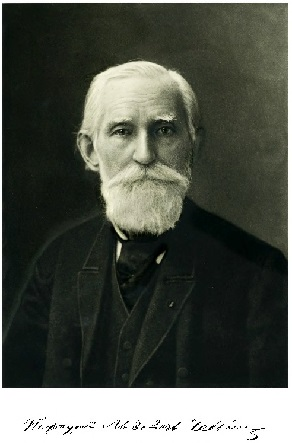To put it short — Pafnutiy Lvovich Chebyshev is our everything in math, he is not unlike Pushkin in that regard.

In addition to being a famous mathematician with major achievements in probability, mechanics, number theory, analysis, and many other fields, he is also viewed as the father of Russian mathematics — a progenitor of an unbroken line of Russian mathematical schools.

In honor of his 200th birthday we present this mini-series of lectures highlighting some of his numerous contributions to the science.

The lectures will be given online using ZOOM, participants are welcome to register here (the notification with the meeting link will be sent after the registration).

This event is a part of the Year of Science and Technology program.

## Program

15.00  Athanase Papadopoulos (Université de Strasbourg)

###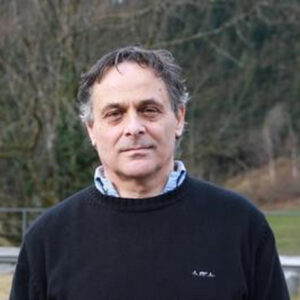16.00  Nikita Kalinin (Saint-Petersburg State University)

### Cartography and mathematics of Chebyshev.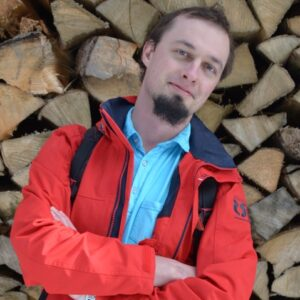17.00  Alisa Sedunova (Saint-Petersburg State University)

### Chebyshev’s contribution to analytic number theory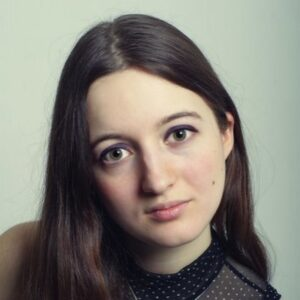18.00  NIck Trefethen (University of Oxford)

###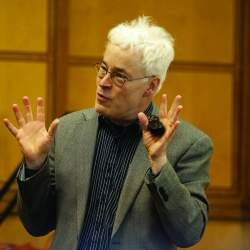19.00  Dmitriy Zaporozhets (Saint-Petersburg State University and PDMI RAS)

### Chebyshev and Probability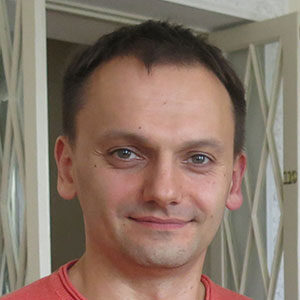It is usual to oppose the mathematical school founded in Saint Petersburg by Pafnuty Lvovich Chebyshev and that of Leonhard Euler. In this talk, I will on the contrary stress on the similarities between the works of the two mathematicians. At the same time, this will give us the occasion to review some of the major achievements of both men and to establish links between their works.

This is a lectures for general audience. Consider a part of a surface (a sphere for example). How to draw a planar map of this surface such that all the angles between curves on this surface would be preserved and the variation of the scale across the map would be minimal? I explain how this is related to conformal maps and explain a proof of Chebyshev’s theorem about such a map in the case of a sphere.

Most of analytic number theory was inspired by the celebrated prime number theorem,which tells us that the number of primes up to x verifies

π(x) = #{p≤x} x/log x

It was conjectured by Legendre in 1797/1798 and later in a more precise shape in 1808, while Gauss has considered that question in 1792/1793.
For a long time there was very little progress towards this the asymptotics, however, in two papers from 1848 and 1850, Chebyshev succeeded in proving a slightly weaker form of the asymptotic, namely, he showed that there are (explicitly given) constants C1, C2 > 0 such that

c x/log x ≤ π (x) ≤ C x/log x.

The above inequalities are called Chebyshev bounds. He also showed that if the limit lim π(x)/(x/ log x) exists as x → ∞, then it must be equal to one.
The prime number theorem was finally proven much later, in 1896, independently by Hadamard and de la Valée-Poussin with the help of complex analytic properties of the Riemann zeta-function. However the ideas of Chebyshev are of extreme importance of are frequently used till today.
In the talk we discuss the proof of Chebyshev bounds, its consequences and how analytic number theorists use them nowadays.

Mathematically, Fourier series (for even periodic functions) and Chebyshev series (for nonperiodic functions) are equivalent. (Did Chebyshev recognize this?) This talk will showcase with Chebfun the extraordinary computational power of Chebyshev polynomials.

In this talk, we will discuss the impact of Chebyshev’s mathematics on Probability theory. For a wide audience with no special mathematical background.

### Registration

The registration is open here.